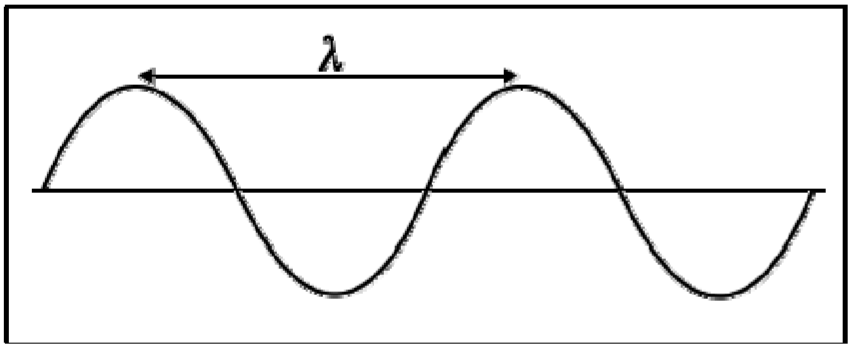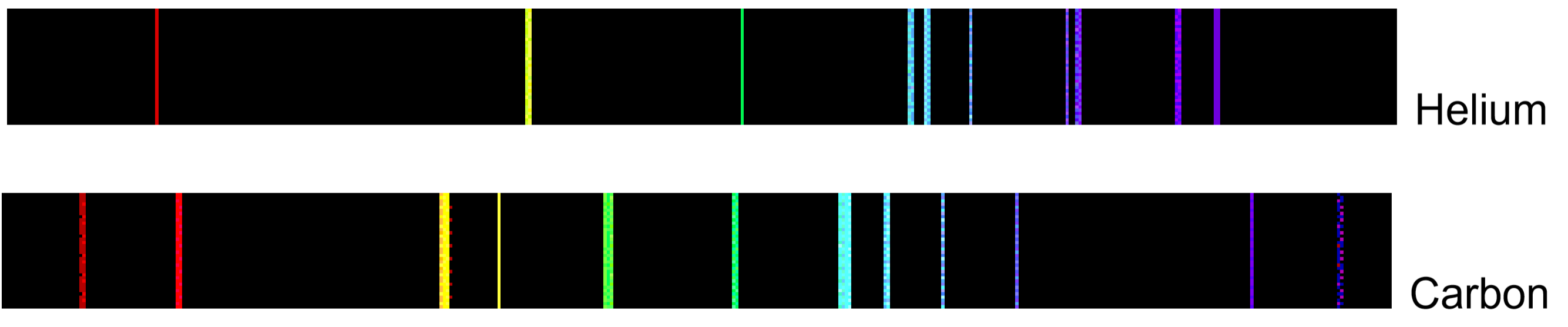# 8: Flame Tests of Metal Cations (Experiment)

$$\newcommand{\vecs}{\overset { \rightharpoonup} {\mathbf{#1}} }$$ $$\newcommand{\vecd}{\overset{-\!-\!\rightharpoonup}{\vphantom{a}\smash {#1}}}$$$$\newcommand{\id}{\mathrm{id}}$$ $$\newcommand{\Span}{\mathrm{span}}$$ $$\newcommand{\kernel}{\mathrm{null}\,}$$ $$\newcommand{\range}{\mathrm{range}\,}$$ $$\newcommand{\RealPart}{\mathrm{Re}}$$ $$\newcommand{\ImaginaryPart}{\mathrm{Im}}$$ $$\newcommand{\Argument}{\mathrm{Arg}}$$ $$\newcommand{\norm}{\| #1 \|}$$ $$\newcommand{\inner}{\langle #1, #2 \rangle}$$ $$\newcommand{\Span}{\mathrm{span}}$$ $$\newcommand{\id}{\mathrm{id}}$$ $$\newcommand{\Span}{\mathrm{span}}$$ $$\newcommand{\kernel}{\mathrm{null}\,}$$ $$\newcommand{\range}{\mathrm{range}\,}$$ $$\newcommand{\RealPart}{\mathrm{Re}}$$ $$\newcommand{\ImaginaryPart}{\mathrm{Im}}$$ $$\newcommand{\Argument}{\mathrm{Arg}}$$ $$\newcommand{\norm}{\| #1 \|}$$ $$\newcommand{\inner}{\langle #1, #2 \rangle}$$ $$\newcommand{\Span}{\mathrm{span}}$$

##### Objectives
• To perform flame tests of metal cations in order to observe their characteristic colors,
• To match the flame colors observed to an appropriate wavelength of visible light, and then perform calculations to determine the frequency and energy of the emitted photons,
• To relate these results to the types of electronic transitions occurring in these elements,
• To practice writing electron configurations for these (and other) elements.

Electromagnetic radiation is composed of perpendicular waves oscillating in the electric and magnetic fields (through space or matter). These waves are characterized by their wavelength (λ) and frequency ($$\nu$$). Wavelength is defined as the distance between successive crests (or troughs) on a wave, and is measured in meters. Frequency is defined as the number of waves that pass a given point every second, and is measured in 1/seconds, or Hertz (Hz).All electromagnetic waves travel at the speed of light ($$c$$), or $$2.998 \times 10^8 m/s$$. The relationship between the wavelength, frequency and speed of an electromagnetic wave is given by the equation:

$c = λ × \nu$

Electromagnetic radiation also occurs as discreet packets of energy (or quanta) called photons. The energy per photon (in Joules) is given by the equation:

$E_{photon} =h×\nu$

Here, $$h$$ is Planck’s constant, which has a value of $$6.626 \times 10^{-34} J \cdot s$$.

Visible light is the most familiar example of electromagnetic radiation. Differences in the wavelengths of visible light are manifested as different colors, shown in the Color Spectrum below (colors can be seen in the PDF document on-line). Other examples of electromagnetic radiation include X-rays, ultraviolet light, infrared light, microwaves and radio waves.So, how does electromagnetic radiation relate to flame tests? Well, when an atom (or ion) absorbs energy, its electrons can make transitions from lower energy levels to higher energy levels. The energy absorbed could be in the form of heat (as in flame tests), or electrical energy, or electromagnetic radiation. However, when electrons subsequently return from higher energy levels to lower energy levels, energy is released predominantly in the form of electromagnetic radiation.

The spacing between energy levels in an atom determines the sizes of the transitions that occur, and thus the energy and wavelengths of the collection of photons emitted:If emitted photons are in the visible region of the spectrum, they may be perceived as lines of different colors (note that photons outside the visible spectrum may also be emitted, but cannot be seen by eye). The result is called a line emission spectrum, and can serve as a ‘fingerprint’ of the element to which the atoms belong. For example, the line spectra shown below for the elements helium and carbon are clearly quite different.Unfortunately, techniques more sophisticated than those used in this lab are required to obtain such line spectra. To the naked eye, when an element is vaporized in a flame (or an electrical discharge) the emission spectrum will appear to be just one color. For example, helium gas when excited by an electrical discharge emits light that appears an orange-peach color. This one color results from a combination of all lines of the emission spectrum, in proportion to their intensities. As many elements will still produce distinctive colors under such conditions, simple flame tests can be used to identify these elements. In fact, flame tests were used to identify elements long before the invention of modern techniques, such as emission spectroscopy.## Procedure

Materials and Equipment

Looped platinum or nichrome wires, wash bottle with distilled water, Bunsen burner, and the following solutions: $$\ce{LiCl}$$ (aq), $$\ce{NaCl}$$ (aq), $$\ce{KCl}$$ (aq), $$\ce{CuCl2}$$ (aq), $$\ce{BaCl2}$$ (aq), $$\ce{CaCl2}$$ (aq).

##### Safety

Exercise appropriate caution when using the Bunsen burner.

Experimental Procedure

This experiment will be performed as an instructor demonstration only.

Your instructor will dip a looped wire into one of the solutions supplied, and then hold it in the Bunsen burner flame. Students will record the dominant flame color observed. The table below contains a list of appropriate colors to choose from. Your instructor will then repeat this for the remaining five solutions, using a fresh looped wire each time.

Analysis: For each metal cation tested, obtain the wavelength of light corresponding to the observed flame color from the table below. Note that the wavelengths supplied here are in nanometers. Using these wavelengths, calculate the frequency and energy of the photons emitted during the flame tests. Finally, answer the questions and perform the exercises as indicated on your Report form.

Dominant Color

Approximate Wavelength (in nm)*

Red

701

Red-Orange

622

Orange

609

Orange-Yellow

597

Yellow

587

Yellow-Green

577

Green

535

Green-Blue

492

Blue

474

Blue-Violet

455

Violet

423

*Wavelength values here are given for the mid- range of the color indicated.

## Pre-laboratory Assignment: Flame Tests of Metal Cations

1. In this lab, you will perform flame tests of several different metal cations. The characteristic colors observed are due to emitted electromagnetic radiation from the excited metal cations.
• In this lab, how do the metal cations become "excited"?
• Circle the correct responses to complete the following statement:

EM radiation is emitted when electrons make transitions from low / high to low / high energy levels.

1. In a flame test, the element Boron emits EM radiation that is predominantly green in color.
• Use the Table in the Procedure to obtain the wavelength of this emitted radiation (in nm). Then convert this wavelength from nm to m.
• Calculate the frequency of this emitted green radiation, in s-1. Show your work.
• Calculate the energy of this emitted green radiation, in J. Show your work.
1. Circle the type of EM radiation that has the property indicated:
• Higher energy: UV or Visible light?
• Longer wavelength: Orange or Blue light?
• Higher frequency: IR or Yellow light?

### Lab Report: Flame Tests of Metal Cations

Experimental Data and Observations

Solution

Dominant Flame Color

Wavelength (nm)

$$\ce{LiCl}$$

$$\ce{NaCl}$$

$$\ce{KCl}$$

$$\ce{CuCl2}$$

$$\ce{BaCl2}$$

$$\ce{CaCl2}$$

Data Analysis

Using the wavelengths recorded above, calculate the corresponding frequencies and photon energies for the emitted radiation observed for each compound tested. Record the results of your calculations in the table below.

Solution

Wavelength (m)

Frequency (s-1)

Photon Energy (J)

$$\ce{LiCl}$$

$$\ce{NaCl}$$

$$\ce{KCl}$$

$$\ce{CuCl2}$$

$$\ce{BaCl2}$$

$$\ce{CaCl2}$$

Show a set of sample calculations for $$\ce{LiCl}$$ only below. Clearly show any equations you have used.

• Wavelength (in m):
• Frequency (in s-1):
• Photon Energy (in J):

#### Questions

1. Complete the following paragraph by circling the correct responses:

In this experiment, the metal cations in the solutions were initially in the (ground, excited) state. When placed in the flame, the metals then (absorbed, emitted) energy as (electricity, heat, EM radiation). When this occurred, electrons made transitions from (low, high) energy levels to (low, high) energy levels. The metals were then in the (ground, excited) state. The electrons in these metals then made transitions from (low, high) energy levels to (low, high) energy levels, resulting in the (absorption, emission) of energy as (electricity, heat, EM radiation).

1. What evidence is there that the colors observed in the flame tests are due to the metals, and not the nonmetals in the compounds tested?
1. Which metal cation was observed to emit radiation with the shortest wavelength?

Compared to the other metals studied, did the radiation emitted by this metal cation (identified above) have

• the highest or lowest frequency?
• the highest or lowest photon energy?

From this, would you conclude that the relationships between the following are direct or inverse?

• wavelength and frequency
• frequency and photon energy
• wavelength and photon energy
1. The energy, wavelength and frequency of an emitted photon are all related to the size of the electronic transition (high → low energy levels) occurring in the metal cation. Based on your observations, in which metal did the smallest electronic transitions occur? Briefly explain your response.
2. When heated in a flame, the element Indium emits electromagnetic radiation with a distinctive indigo blue color (the name indium is derived from the word indigo). The emitted photons that give rise to this color have energies of $$4.405 \times 10^{-19} J$$. Calculate the wavelength of this radiation in nanometers.

#### Exercise

In the Bohr Model of the atom, electrons occupy fixed orbits around the nucleus called energy levels. However in the Quantum Mechanical Model of the atom, electrons occupy orbitals. Orbitals are grouped by size and shape into shells and subshells (or, levels, and sublevels). Electron configurations and orbital diagrams are used to show the arrangement of electrons in shells (levels), subshells (sublevels) and orbitals for specific atoms.

Write complete electron configurations and abbreviated orbital diagrams for each of the elements given below. Circle the valence electrons in your complete electron configurations.

Chlorine

complete configuration:

abbreviated orbital diagram:

Tin

complete configuration:

abbreviated orbital diagram:

Selenium

complete configuration:

abbreviated orbital diagram:

Cobalt

complete configuration:

abbreviated orbital diagram:

Boron

complete configuration:

abbreviated orbital diagram:

Bismuth

complete configuration:

abbreviated orbital diagram:

Magnesium

complete configuration:

abbreviated orbital diagram:

8: Flame Tests of Metal Cations (Experiment) is shared under a CC BY-NC license and was authored, remixed, and/or curated by Santa Monica College.